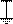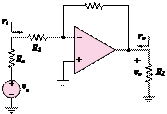### Create an Account

Already have account?

### Forgot Your Password ?

Home / Questions / The amplifier shown in Figure P829 has a signal source a load and one stage of amplification with

# The amplifier shown in Figure P829 has a signal source a load and one stage of amplification with

The amplifier shown in Figure P8.29 has a signal source, a load, and one stage of amplification with

RS  = 2.2 k2          R1  = 1 k2

RF  = 8.7 k2         RL  = 20 2

Motorola MC1741C op-amp:

r= 2 M2             r= 25 2 μ = 200,000

In a first-approximation analysis, the op-amp parameters given above would be neglected and the op-amp modeled as an ideal device. In this problem, include their effects on the input resistance of the amplifier circuit.

Vbatt

_

+v1(t)

vout(t)

a.    Derive an expression for the input resistance vi/ii

including the effects of the op-amp.

b.    Determine the value of the input resistance including the effects of the op-amp.

c.    Determine the value of the input resistance assuming the op-amp is ideal.

Figure P8.26RF

Jun 18 2020 View more View Less

#### Answer (Solved)Subscribe To Get Solution